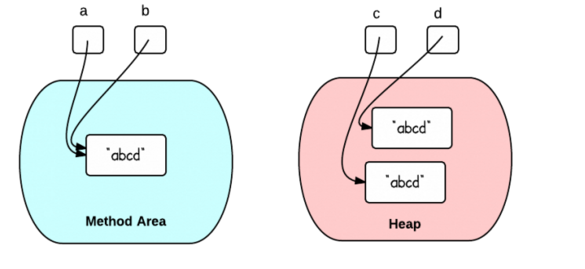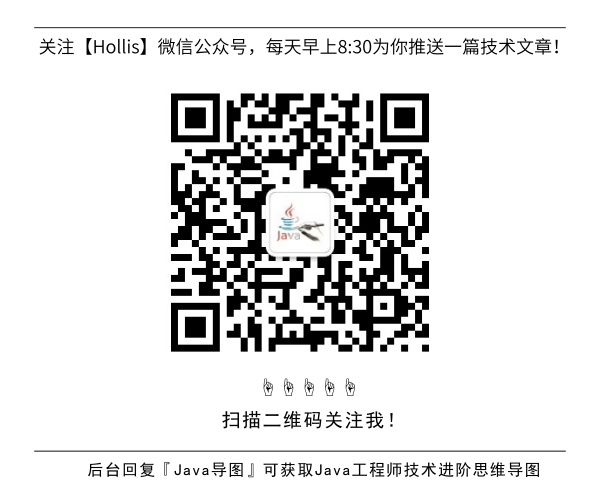# 该如何创建字符串，使用” “还是构造函数？

GitHub 19k Star 的Java工程师成神之路，不来了解一下吗！

``````String x = "abc";
String y = new String("abc");
``````

## 双引号 vs 构造函数

``````String a = "abcd";
String b = "abcd";
System.out.println("a == b : "+(a == b)); // true
System.out.println("a.equals(b) : "+(a.equals(b))); // true
``````

`a== b`等于`true` 是因为x和y指向方法区中同一个字符串常量，他们的引用是相同的（==比较的是引用）。

``````String c = new String("abcd");
String d = new String("abcd");
System.out.println("c == d : "+(c == d)); // false
System.out.println("c.equals(d) : "+(c.equals(d))); // true
``````

`c== d`等于`false` 是因为c和d指向堆中不同的对象。不同的对象拥有不同的内存引用。## 运行时字符串驻留

``````String c = new String("abcd").intern();
String d = new String("abcd").intern();
System.out.println("c == d : "+(c == d)); // true
System.out.println("c.equals(d) : "+(c.equals(d))); // true    （JDK1.7）
``````

(全文完)### 评论 1

1. #1

第二幅图中，在 JAVA 1.7，堆中存的不是 abcd ，而是一个引用指向字符串常量池中的 abcd 吧？

北仑色5年前 (2018-03-30)回复

## HollisChuang's Blog

• 微信咨询
• 去评论
• 回顶
###### 回顶部